# Team:Groningen/Software

iGem Groningen 2018#### Introduction

Buffy the Bufferbot is designed as a example of how Artificial Intelligence can be used to make work in the lab easier. The objective of the robot is to get an unknown buffer and add enough base or acid until it reaches the right pH. The goal is to make a plug-and-play, low cost robot, to lower the efforts of the people in the lab. For now Buffy only works in simulation, and is only used for demonstration, but in the future, we hope that Buffy will have a body so it can help people all over the world.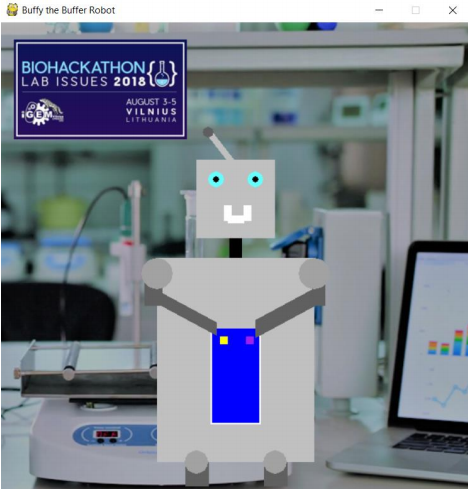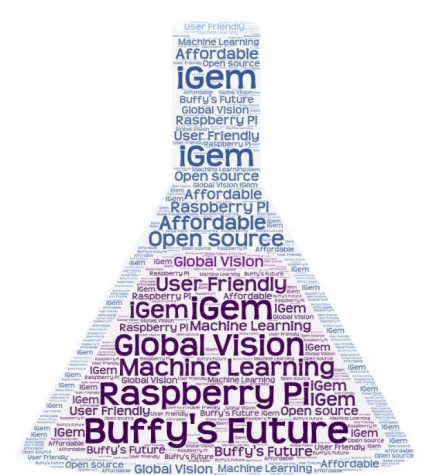#### How to use the simulation

As buffy is properly packaged, it can be installed directly from the source by running “pip install git+https://github.com/Ezmagon/buffy” in a command line terminal. A wrapper script to run the buffy simulation is then added to your path and can be run using “buffy_run.py”. The program opens a TKinter window to accept the goal pH as input. This is the only input that is needed as buffy is able to derive all the other system parameters by itself. When computer vision is pressed in the next window, it starts the simulation of how the finished computer vision will be able to automatically read the current pH of the buffer from an existing pH meter. The system incrementally shows how the algorithm approaches the correct area of the image and reads the numbers. When a key is pressed, the simulation windows opens.

#### Results

The simulation window shows the underlying (real) buffer pH curve in orange that is being simulated. Just like in the future hardware version of buffy, it doesn’t know this curve beforehand. Buffy will start to add acid or base depending on whether the pH has to increase or decrease. The new pH of the buffer is recorded. As buffy records more data points, it is able to fit a third order polynomial to the data points, shown as the green line. The fit gets progressively more accurate as more data points are gathered. Despite the addition of random noise to the data points, buffy is able to accurately predict the real underlying pH curve of the buffer. Using this fit it is possible to make increasingly accurate predictions of how much acid or base to add to the solution, drastically reducing the time required to adjust a buffer manually.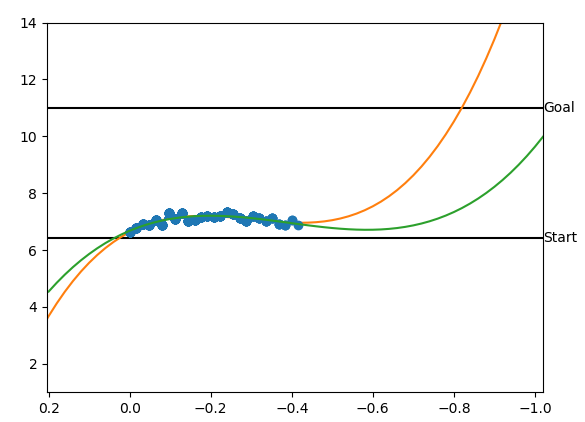# A look inside the brain of a robot!

Buffy uses a feedback loop to calculate which should be added, acid or base. First it observes the pH meter by a computer vision module. After this, it processes this information. Based on it's knowledge, it then makes a choice on what action to take. In the future, the consequences from these actions can be used to train the machine.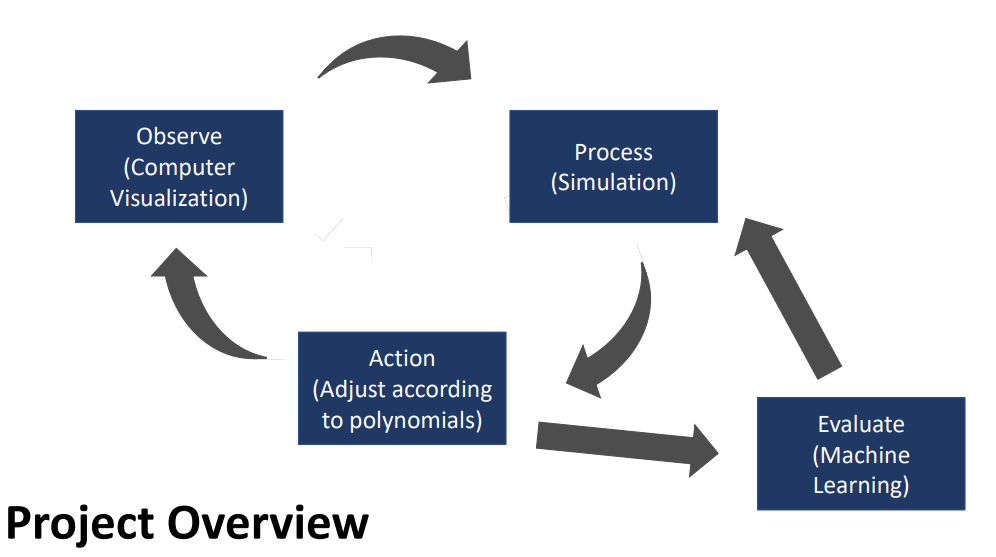#### The simulation

To be able to properly simulate the pH curve of the buffer, the “real” buffer pH has to be approximated by a continuous function. The well established Henderson-Hasselbalch equation (pH = pKa + log10 ([A-]/[HA])) was used to generate data points within the range of the buffer. However, this function tends to infinity as one of the buffer components approaches zero. Therefore the rest of the data points were linearly approximated. A third order polynomial was fit to the data points to yield a continuous function that could be used to approximate the pH range across the entire pH range.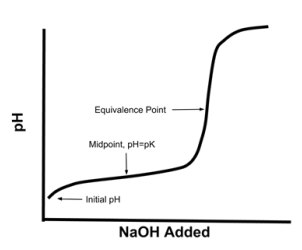#### Observe

The computer vision algorithm uses different techniques to find the number on the screen. The amount of deep learning is minimized, as we want to make Buffy as memory efficient as possible. (1) First , the algorithm uses a Gaussian blur to smoothen out the noise in the camera view. (2) After this, the Canny Edge Detection algorithm is used, and this way the edges are clear for Buffy. (3) Now, the contours are found, and the biggest ones are marked. After this, it focuses on the biggest contour to find the pH meter. (4) After this, the process is repeated to find the screen of the pH meter. Then, after the Canny Edge Detection is used, (5) Buffy looks for contours of the right size to find the numbers. (6) If the numbers are found, the area of interest is taken out and put into a Neural Network trained on recognizing ResNet numbers. This returns the number of the pH meter. We are still working to optimize this process.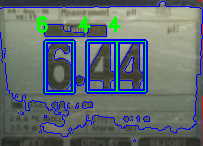#### Predict the polynomial of the buffer

To predict the polynomial of the buffer, Buffy has to predict where the buffer is, as the pH curve is not linear. Therefore we gave it knowledge of the buffer curve, which is that all buffers are polynomials to the 3rd degree. Therefore, Buffy expects a line in the form of ax3 + bx2 + cx + d = y. To give the user insight in the knowledge of Buffy, the line is projected over the real line, and over time we can see it is learning, and coming closer to the right point.